# Proof Manager

The Proof Manager is responsible for constructing proofs and maintaining existing proofs associated with proof obligations.

## Overview

Proof obligations are generated by the proof obligation generator and have the form of sequents.

Sequents are proved using proof rules.

The Proof Manager architecture is separated into two parts: extensible part and static part. The extensible part is responsible for generating individual proof rules. The static part is responsible for putting proof rules together to construct and manage proofs. We call components that generate valid proof rules reasoners.

The basic reasoning capabilities of the Proof Manager can be extended by adding new reasoners. A reasoner may implement a decision procedure for automated proof, or a derived rule schema for interactive proof.

By applying the generated proof rules by different reasoner, the Proof Manager builds a (partial) proof for an proof obligation by constructing proof trees.

In order to encapsulate frequently used proof construction and manipulation steps, the Proof Manager provides the concept of tactics. They provides high-level strategic proof manipulations. Adding new tactics is the second possibility for extending the Proof Manager.

## Proof Rules

In its pure mathematical form, a proof rule is a tool to perform formal proof and is denoted by: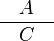$\frac{\quad A\quad}{C}$

where A is a (possibly empty) list of sequents:the antecedents of the proof rule; and C is a sequent: the consequent of the rule. And we interpret the above proof rule as follows: The proofs of each sequent of A together give a proof of sequent C.

### Representation

In Rodin, the representation for proof rules are more structure not only to reduce the space required to store the rule, but more importantly to support proof reuse.

A rule in Rodin contains the following:

• used goal A used goal predicate.
• used hypotheses The set of used hypotheses.
• antecedents A list of antecedents (to be explain later).
• reasoner the reasoner used to generate this proof rule (See reasoners).
• reasoner input the input to the reasoner to generate this proof rule (See reasoners).

Each antecedent of the proof rule contains the following information:

• new goal A new goal predicate.
• added hypotheses The set of added hypotheses.

With this representation, a proof rule in Rodin corresponding to a proof schema as follows: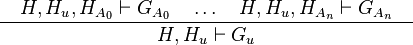$\frac{\quad H, H_u, H_{A_0} \vdash G_{A_0} ~~~\ldots~~~ H, H_u, H_{A_n} \vdash G_{A_n}\quad}{H, H_u \vdash G_u}$

Where:

•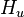$H_u$ is the set of used hypotheses
•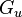$G_u$ is the used goal
•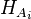$H_{A_i}$ is the set of added hypotheses corresponding to the ith antecedent.
•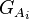$G_{A_i}$ is the new goal corresponding to the ith antecedent.
•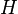$H$ is the meta-variable that can be instantiated.

### Applying Proof Rules

Given a proof rule of the form mentioned above, the following describes how to apply this rule to an input sequent. The result of this process is a list of output sequents if successful or null otherwise.

• The rule is NOT applicable if the goal of the sequent is not exactly the same as the used goal or any of the used hypotheses is not contained in the set of hypotheses of the input sequent.
• In the case of applicable, the antecedent sequents are returned. The goal of each antecedent sequent is the new goal correspondingly. The hypotheses of each antecedent sequent is the union of the old hypotheses and added hypotheses of the corresponding antecedent.

TODO

## Reasoners

Reasoners are responsible for generating Proof Rules. The input of a reasoner is:

1. A sequent.

2. Optional input (e.g. a predicate in the case of the Cut Rule).

The output of the reasoner (in case of successful) is a proof rule. This proof rule is trusted by the Proof Manager to built the proof.

An important point about reasoners is that the internal workings of them are not visible to the other part of the Proof Manager. It is only required that they satisfy the following requirements:

1. Logically Valid A generated proof rule must be valid (i.e. can be derived) in the mathematical logic.

2. Replayable A reasoner must work deterministically, i.e. given the same input, a reasoner must generate the same proof rule every time.

### Examples of Reasoners

TODO

TODO Explain "succeed".

## Proof Trees

Proof trees are recursive structure based on Proof Tree Nodes. Each node has three components:

1. sequent A sequent.

2. rule A proof rule (possibly null)

3. children A list of proof tree nodes (possibly null or empty).

A proof tree is a proof tree node where its sequent corresponding to the proof obligation.

A proof tree node has different statuses:

a. Pending No rule applied to this node, i.e. rule is null. In this case, children must be null.

b. Non-pending Has a rule applied to this node. In this case, children contains the list of child proof tree nodes.

We now review the constraints on the proof trees, follows by different operations on the proof trees.

### Constraints on Proof Trees

A proof tree is valid if all its proof tree nodes are valid.

A pending proof tree node is valid if its children is null

A non-pending proof tree node is valid if:

• It's children is not null.
• rule (which is not null) is applicable to the sequent.
• children corresponding to the result of the application of rule to sequent.
• Each of the children proof tree nodes is valid.

### Operations on Proof Trees

• Construction Create an initial proof tree corresponding to an input sequent. The resulting proof tree containing a single root node. This root node is a pending node with corresponding to an input sequent.
• Rule application A proof tree grows when a rule applies to one of its pending node. The input rule is first check for applicability to the sequent corresponding to the pending node. If successful, the rule is attach to the node, then a new children nodes are attached according to the outcome of the application of the rule.
• Pruning A proof tree can be pruned at any of its proof tree nodes. The rule and the children associated with that node will be removed (reset to null).
• Getting the list of pending nodes A list of pending nodes can be computed for any proof tree.
• Checking if the proof tree is complete A proof tree is complete if it does not contain any pending proof tree node.

TODO

## Tactics

Tactics provide an easier way to construct and manage proof search and manipulation. They provide calls to the underlying reasoners or other tactics to modify proofs.

The input of a tactic is a proof tree node which will be used as the point of application. The output of a tactic is a boolean to indicate if it is successful or not.

We will describe below some examples of tactics.

TODO Clarify "succeed" or "failure".

### Basic Tactics

They are those tactics that make change to the proof tree only at the point of application.

• Prune A direct application of the pruning facility providing by the proof tree. The tactic is successful if the input node are non-pending.
• Rule Application Tactics of this class provide a wrapper around a proof rule (See Proof Rules). The tactic is successful if the proof rule is successful applied to the input node.
• Reasoner Application Tactics of this class provide a wrapper around a reasoner (See Reasoner). The tactic is successful if the reasoner is successful applied to the input node.

### Tactical Tactics

They are those tactics that are constructed from existing tactics. They indicate different strategic or heuristic decisions.

• Apply on All Pending Apply a specific sub-tactic to all pending nodes of the point of application. The tactic is successful if the sub-tactic is successful on one of the pending nodes.
• Repeating Repeating a specific sub-tactic to the point of application until it fails. The tactic is successful if the sub-tactic is successful at least once.
• Composing Sequential compose a list of sub-tactics to apply to the point of application. The tactic is successful if one of the sub-tactic is successful.

More complex proof strategy can be constructed by recursively applying the above tactical tactics.